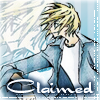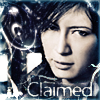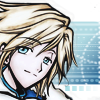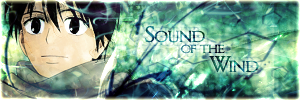Forces acting on a spinning object? Link | byon 2010-04-27 06:19:39 (edited 2010-04-27 06:19:58) If there was an object attached to a string and then spun in a circle horizonatlly, what would the full body diagram look like? I know there is centripetal force and tension involved however which direction would they go in? Also there would be vertical forces involved (as this is supposed to represent a non-idealistic situation) so which ones would be pressent and which direction would they be in?Re: Forces acting on a spinning object? Link | by desertfox on 2010-04-28 10:09:50 well, the force on the object is: force due to speed which is tangential to the circle force due to the string (or centripetal force that makes it go in circle) which is directed to the point of origin of string force due to gravity (which acts downwards) assuming you're spinning the object in a circle in a plane parallel to the ground, then gravity will make the object fall below the plane. However, the upward component of centripetal force (which is directed to the point of origin of string) will counteract it to form an equilibrum, hence the object doesn't fall down.

 Re: Forces acting on a spinning object? Link | byon 2010-04-28 11:09:48 thanks ^_^